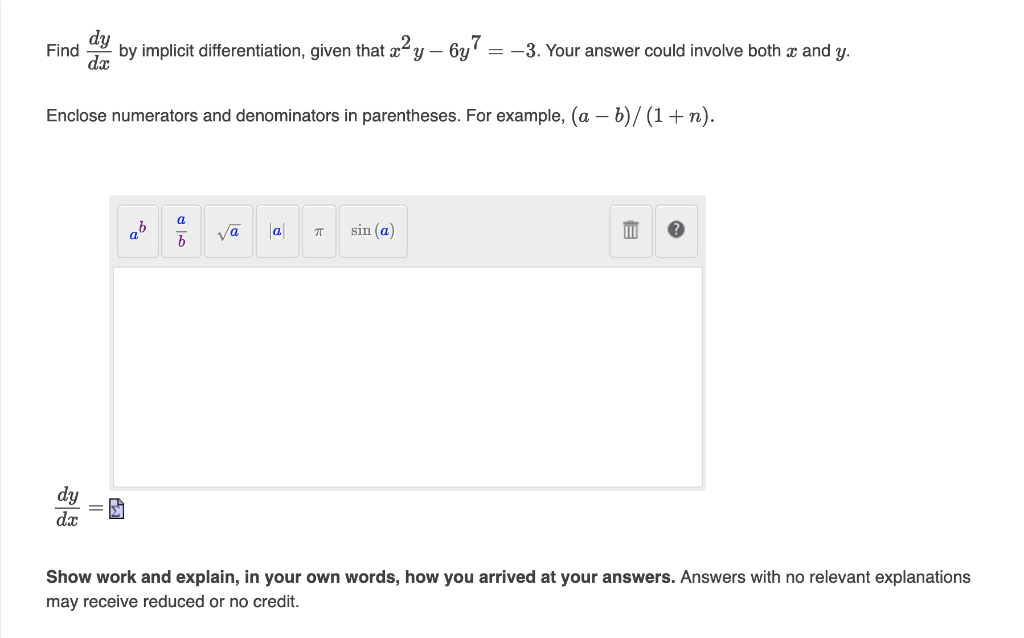# Question i need help with this please Find $$\frac{d y}{d x}$$ by implicit differentiation, given that $$x^{2} y-6 y^{7}=-3$$. Your answer could involve both $$x$$ and $$y$$. Enclose numerators and denominators in parentheses. For example, $$(a-b) /(1+n)$$. $\frac{d y}{d x}=\text { 㐫 }$ Show work and explain, in your own words, how you arrived at your answers. Answers with no relevant explanations may receive reduced or no credit.i need help with this please
Transcribed Image Text: Find $$\frac{d y}{d x}$$ by implicit differentiation, given that $$x^{2} y-6 y^{7}=-3$$. Your answer could involve both $$x$$ and $$y$$. Enclose numerators and denominators in parentheses. For example, $$(a-b) /(1+n)$$. $\frac{d y}{d x}=\text { 㐫 }$ Show work and explain, in your own words, how you arrived at your answers. Answers with no relevant explanations may receive reduced or no credit.
Transcribed Image Text: Find $$\frac{d y}{d x}$$ by implicit differentiation, given that $$x^{2} y-6 y^{7}=-3$$. Your answer could involve both $$x$$ and $$y$$. Enclose numerators and denominators in parentheses. For example, $$(a-b) /(1+n)$$. $\frac{d y}{d x}=\text { 㐫 }$ Show work and explain, in your own words, how you arrived at your answers. Answers with no relevant explanations may receive reduced or no credit.&#12304;General guidance&#12305;The answer provided below has been developed in a clear step by step manner.Step1/1solution; given that the equation is $$\mathrm{{x}^{{2}}{y}-{6}{y}^{{7}}=-{3}}$$now we implicitely differentiate it w,r,t,x that is $$\mathrm{\frac{{d}}{{{\left.{d}{x}\right.}}}{\left({x}^{{2}}{y}-{6}{y}^{{7}}\right)}=\frac{{d}}{{{\left.{d}{x}\right.}}}{\left(-{3}\right)}}$$\( \mathrm{{y}\frac{{d}}{{{\left.{d}{x}\right.}}}{\left({x}^{{2}}\right)}+{x}^{{2}}\frac{{d}}{{{\left.{d}{x}\right.}}}{\left({y}\right)}-\frac{{d}}{{{\left.{d}{x}\right.}}}{\ ... See the full answer